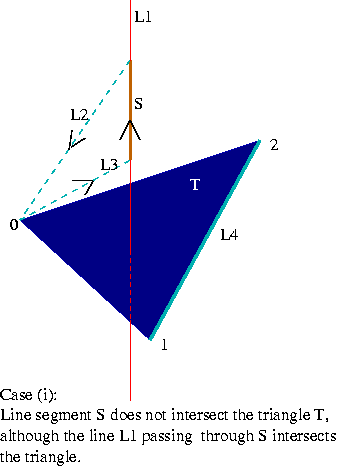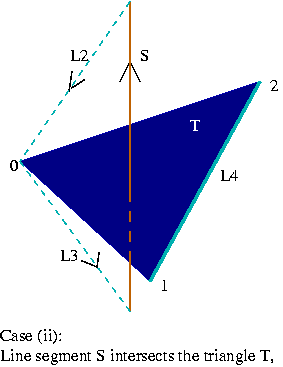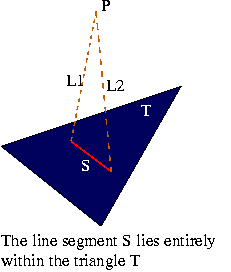Intersection between a Line Segment and a Triangle

Problem:
Input: The line segment L is given as input in the form of two end points. The input for triangle T is given in the form of three points (its three vertices v0, v1 and v2).
Output: We return the type of intersection (see the lists below) and the vertices or the edges involved.

 Triangle:                     proper intersection                     no intersection                     intersection at a vertex                     intersection on an edge                     coplanar and no intersection                     coplanar and intersection at vertex                     intersection through two edges                     intersection contains an edge                     intersection through one vertex and an edge Segment:                     proper intersection                     no intersection                     intersection at a vertex

Representation:
We use the plucker coordinates to solve this problem. We compute the side-operator of the line  L with the three oriented lines e1(v0 to v1), e2(v1 to v2) and  e3(v2 to v0) supporting the edges of the triangle.

S1 = side-operator(L,e1)
S2 = side-operator(L,e2)
S3 = side-operator(L,e3)

This problem is an extension of the problem of computing the INTERSECTION BETWEEN A LINE AND A TRIANGLE. In solving this problem we make use of the earlier algorithm and build upon it.

Algorithm:
(1) Check for the intersection between the line containing the line segment and the triangle. Either

a) The line and the triangle are not coplanar or,
b) The line and the triangle are coplanar.

(2) For case (a) if the intersection between the triangle and line returns "proper intersection", "intersection at a vertex" or "intersection on an edge" then we need to check wheter the line segment intersects the triangle or not. This is done as follows:

The two possible cases are shown below.Constructing lines L2, L3 and L4:
L2 and L3 are directed lines passing through the end points of the line segment and a common vertex of the triangle. We can choose the common vertex as follows:

If the intersection between the triangle and the line returns "proper intersection" or "intersection on an edge" then we are free to choose any of the three vertices of the triangle for the construction of L2 and L3 (this ensures that L2 and L3 are defined when an endpoint of the line segment coincides with a vertex of a triangle.

If the intersection between the triangle and the line returns "intersection at a vertex" then we can choose any of the other two vertices for constructing L2 and L3.

L2 is the oriented line from one end point of the segment S to the common vertex (labeled 0 in the figure) and L3 is the oriented line from the common vertex to the other end point of the segment S.

Line L4 is the line passing through the other two vertices of the triangle (or the line containing the edge opposite to the chosen common vertex).

Let,
s1 = side-operator(L4,L3)
s2 = side-operator(L4,L2)

If s1 and s2 have opposite signs, the line segment does not intersect the triangle. If they have the same sign then,
the line segment intersects the triangle. If one of them is zero , the triangle and the line segment intersect  at one endpoint of the line segment.

(3) For the case(b) when the line and triangle are coplanar, we find the intersection between the line and the triangle. If it returns "coplanar and no intersection" then there is no intersection between the line segment and triangle. Otherwise we have to determine wheter the line segment intersects any of the three edges of the triangle. The problem reduces to finding the intersection between two line segments.

(4) One case which still remains, is when the line segment and the triangle are coplanar and the line segment lies entirely within the triangle:Take a point P outside the plane of the triangle and construct the lines L1 and L2. These lines should both intersect the face of the triangle i.e, we should get "proper intersection" for the line and triangle intersection.